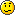## Parabxot para AmiBroker

Mensajes: 11976
Contactar:

### Parabxot para AmiBroker

Aquí tenéis el indicador en formato AFL del Parabxot de Dennis Meyers.

Código: Seleccionar todo

``````// Downloaded From www.WiseStockTrader.com
//------------------------------------------------------------------------------
//
//  Formula Name:    ParabXO
//  E-mail:          [email protected]
//  Origin:
//  Keywords:
//  Level:           medium
//  Flags:           indicator
//  Formula URL:     http://www.amibroker.com/library/formula.php?id=448
//  Details URL:     http://www.amibroker.com/library/detail.php?id=448
//
//------------------------------------------------------------------------------
//
//  This is an enhancement of the famous Parabolic SAR indicator by Welles
//  Wilder. For more details see the remarks below.
//
//------------------------------------------------------------------------------

/////////////////////////////////
// ParabXO implemented in AFL.
//
// The code below relies heavily on the AFL code for the
// Parabolic SAR by Tomasz Janeczko in the AB library
//
// Application: Drag & Drop.
//
// Aside from making the Accelerator Factor and its maximum
// value changeable via the Param() function I made 2 enhancements
// by some simple additional coding that were introduced by
// Dennis Meyers in an article in the S&C 4/1995 issue:
//
// 1. The start value of the AF can be set independently; thus you
//    can make the indicator react considerably faster.
// 2. The ParabXO does not reverse unless penetrated
//    by a specified amount (called "Crossover threshold in %" below)
//    thus preventing too many whipsaws. It can be set to 0 if
//    you don't want to use this modification. Please note that
//    in Meyers' article he used an absolute number whereas a
//    percentage makes more sense in my humble opinion.

// Written by: Thomas Ludwig

acc=Param("Acceleration factor",0.02,0.01,0.05,0.01);
af_start=Param("Starting AF value",0.02,0.01,0.05,0.01);
af_max=Param("Maximum AF value",0.2,0.1,0.3,0.01);
Ct=Param("Crossover threshold in %",1,0,3,0.5);
Ct1=Ct/100;

IAF = acc;
MaxAF = af_max;     // max acceleration

psar = Close;		// initialize
long = 1;        // assume long for initial conditions
af = af_start;   // starting value of the acelleration factor
ep = Low[ 0 ];   // init extreme point
hp = High [ 0 ];
lp = Low [ 0 ];

for( i = 2; i < BarCount; i++ )
{
if ( long )
{
psar [ i ] = psar [ i-1 ] + af * ( hp - psar [ i-1 ] );
}
else
{
psar [ i ] = psar [ i-1 ] + af * ( lp - psar [ i-1 ] );
}

reverse =  0;
//check for reversal
if ( long )
{
if ( Low [ i ] < psar [ i ] * (1-Ct1) )
{
long = 0; reverse = 1; // reverse position to Short
psar [ i ] =  hp;       // SAR is High point in prev trade
lp = Low [ i ];
af = af_start;
}
}
else
{
if ( High [ i ] > psar [ i ] * (1+Ct1) )
{
long = 1; reverse = 1;        //reverse position to long
psar [ i ] =  lp;
hp = High [ i ];
af = af_start;
}
}

if ( reverse == 0 )
{
if ( long )
{
if ( High [ i ] > hp )
{
hp = High [ i ];
af = af + IAF;
if( af > MaxAF ) af = MaxAF;
}

if( Low[ i - 1 ] < psar[ i ] ) psar[ i ] = Low[ i - 1 ];
if( Low[ i - 2 ] < psar[ i ] ) psar[ i ] = Low[ i - 2 ];
}
else
{
if ( Low [ i ] < lp )
{
lp = Low [ i ];
af = af + IAF;
if( af > MaxAF ) af = MaxAF;
}

if( High[ i - 1 ] > psar[ i ] ) psar[ i ] = High[ i - 1 ];
if( High[ i - 2 ] > psar[ i ] ) psar[ i ] = High[ i - 2 ];

}
}
}

//Plot( Close, "Price", colorBlack, styleCandle );
Plot( psar, _DEFAULT_NAME(), ParamColor( "Color", colorRed ), styleDots | styleNoLine | styleThick );
``````
"Los sistemas de trading pueden funcionar en ciertas condiciones de mercado todo el tiempo, en todas las condiciones de mercado en algún momento del tiempo, pero nunca en todas las condiciones de mercado todo el tiempo."
davidx
Mensajes: 1

### Re: Parabxot, Un Parabólico Mejorado

El código de Amibroker del primer mensaj es el mismo que el que pones en Tradingview?

No me funciona, a ti sí?Muchas gracias